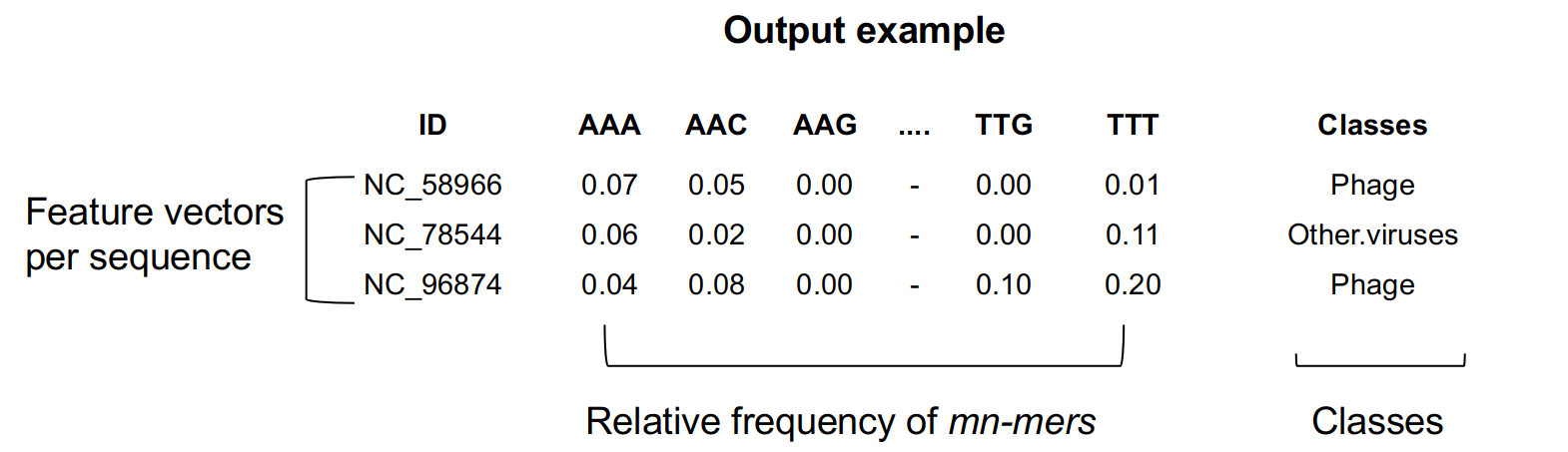# Conditional frequency distribution

The (m,n)-mer R package was created to summarize biological data into numerical characteristics. It reads a FASTA file and generates a table describing the conditional frequency distribution of the selected (m,n)-mer in the sequences. This output is combined with class information to generate the feature matrix for classification.

(m,n)-mers are an alternative for k-mers (Figure 1). We proposed the replacement of the unconditional k-mer frequency by the conditional frequency, which represents the relative frequency of the n-mer conditioned to f m-mer that precedes it. For more details and performance comparison, please see Andrade et al., 2022 (in press).

Fig 1. Comparing k-mer to mn-mer relative frequency.

According to Figure 2, the k-mers are represented as (0,k) and the mn-mers as (m,n).

Fig 2. Numeric representation.

The output table (Figure 3) includes the fasta file accession numbers as an ID column, the relative frequency of mn-mers up to 4^k columns, and class information.Fig 3. Output example.

## Dependencies

``The package needs R(>= 4.2.0), Biostrings(>= 3.1) and Utils(>= 2.0.0).``

## Installation

``````library(devtools)

install_github("labinfo-lncc/mnmer", ref="main")``````

## Quick Start: Running (m,n)-mer on example dataset

``````library("mnmer")
dir <-system.file("extdata", package="mnmer")``````

Assume we need to distinguish between viruses that infect human and viruses that exclusively infect plants.

The `readNumFASTA` function employs Biostrings for reading FASTA files into the R system. It enables users to limit the number of sequences loaded, select sequences at random, and set a non-ACTG base cutoff percentage.

The parameters are:

`FASTAfile` = It could be a multiFASTA.

`size` = Number of sequences to be loaded.

`rand` = Select sequences randomly or not. Set TRUE or FALSE

`pni` = Percentage of non-ACTG (default = 0.20)

As default, all sequences more than 20% of N + IUPAC bases will be removed from further analysis given the little informative nature of those bases. If the user would like to accept these sequences, the `pni` parameter should be set to 0.00. The `readNumFASTA` function returns an DNAStringSet data structure, used by the `mnmer` in further analysis. To learn more about DNAStringSet please check Biostrings documentation.

``````human <-readNumFASTA((file.path(dir, "human_vir.fasta.gz")), 1000,TRUE,0.50)

#### Producing k-mers

The `mnmer` function generates the feature matrix using conditional probability from DNAStringSet objects. This function can generate both k-mers and mn-mers. The parameter `m` is set to choice for k-mer generation, while the parameter `n` is set to zero. Considering that the k-mers are conditioned to zero bases.

``````human_02mer <- mnmer(human,2,0)
plant_02mer <- mnmer(plant,2,0)``````

#### Producing (m,n)-mers

The `m` and `n` parameters are chosen by the user for mn-mer creation. For instance, `m = 1` and `m = 1` yield the (1,1)-mer, in which one base is conditioned on the frequency of one preceding base.

``````human_21mer <- mnmer(human,2,1)
plant_21mer <- mnmer(plant,2,1)``````

For classification outside of the mnmer program, we utilize the (1,1)-mer feature matrices. Here’s a real-world example of code:

``````
library(data.table)
library(caret)
library(MLeval)

classes <- replicate(nrow(human_21mer), "human.vir")
featureMatrix_human <- cbind(human_21mer,classes)
classes <- replicate(nrow(plant_21mer), "plant.vir")
featureMatrix_plant <- cbind(plant_21mer,classes)

featureMatrix <- rbind(featureMatrix_human, featureMatrix_plant)
featureMatrix <- subset(featureMatrix, select = -c(seqid))
train_index <- createDataPartition(featureMatrix\$classes, p=0.8, list=FALSE)
train <- featureMatrix[train_index, ]
test <- featureMatrix[-train_index, ]
control <- trainControl(method="cv", summaryFunction=twoClassSummary, classProbs=T, savePredictions = T)
roc <- train(classes ~ ., data=train, method="rf", preProc=c("center"), trControl=control)
res <- evalm(roc) # Make the ROC plot``````

This classification produces the metrics shown below:

Metrics Value
AUC 1.0000
ROC 1.0000
Sensibility 0.9500
Specificity 1.0000

## Work in progress

If you have any queries or find a bug, please submit an issue on GitHub or email atrv@lncc.br.